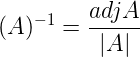GATE | GATE CS 2019 | Question 16

Let X be a square matrix. Consider the following two statements on X.

I. X is invertible
II. Determinant of X is non-zero

Which one of the following is TRUE?
(A) I implies II; II does not imply I
(B) II implies I; I does not imply II
(C) I does not imply II; II does not imply I
(D) I and II are equivalent statements

Explanation: Since, inverse of a matrix is defined as,So, if denominator or divisor (i.e., determinant of matrix, |A|) is zero, then inverse of matrix (i.e., (A)-1) can not be defined.

That means if inverse of matrix (i.e., (A)-1) exists, then it means that denominator (i.e., determinant of matrix, |A|) is non-zero.

A square matrix is Invertible if and only if its determinant is non-zero.

Hence, both given statements are equivalent.

So, option (D) is correct.

Quiz of this Question

My Personal Notes arrow_drop_up

Article Tags :

Be the First to upvote.

Please write to us at contribute@geeksforgeeks.org to report any issue with the above content.